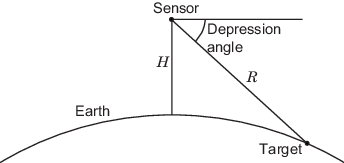# depressionang

Depression angle of surface target

## Syntax

```depAng = depressionang(H,R) depAng = depressionang(ht,r,MODEL) depAng = depressionang(H,R,MODEL,Re) depAng = depressionang(___,'TargetHeight',TGTHT) ```

## Description

`depAng = depressionang(H,R)` returns the depression angle from the horizontal at an altitude of `R` meters to surface targets. The sensor is `H` meters above the surface. `R` is the range from the sensor to the surface targets. The computation assumes a curved earth model with an effective earth radius of approximately 4/3 times the actual earth radius.

`depAng = depressionang(ht,r,MODEL)` specifies the earth model used to compute the depression angle. `MODEL` is either `'Flat'` or `'Curved'`.

`depAng = depressionang(H,R,MODEL,Re)` specifies the effective earth radius. Effective earth radius applies to a curved earth model. When `MODEL` is `'Flat'`, the function ignores `Re`.

`depAng = depressionang(___,'TargetHeight',TGTHT)` specifies the target height, `TGTHT` above the surface as either a scalar or a vector. If any combination of `H`, `R`, and `TGTHT` are vectors, then the dimensions must be equal. `r` must be greater than or equal to the absolute value of the difference of `ht` and `TGTHT`.

## Input Arguments

 `H` Height of the sensor above the surface, in meters. This argument can be a scalar or a vector. If both `H` and `R` are nonscalar, they must have the same dimensions. `R` Distance in meters from the sensor to the surface target. This argument can be a scalar or a vector. If both `H` and `R` are nonscalar, they must have the same dimensions. `R` must be between `H` and the horizon range determined by `TGTHT`. `MODEL` Earth model, as one of | `'Curved'` | `'Flat'` |. Default: `'Curved'` `Re` Effective earth radius in meters. This argument must be a positive scalar value. You can used `effearthradius` to compute the effective radius. Default: `effearthradius`, which is approximately 4/3 times the actual earth radius `TGTHT` Target height above surface, specified as a scalar or vector. If any combination of `H`, `R`, and `TGTHT` are vectors, then their sizes must be equal. `R` must be greater than or equal to the absolute value of the difference of `H` and `TGTHT`. A surface target has a `TGTHT` of zero. Default: `0`

## Output Arguments

 `depAng` Depression angle, in degrees, from the horizontal at the sensor altitude toward surface targets `R` meters from the sensor. The dimensions of `depAng` are the larger of `size(ht)` and `size(r)`.

## Examples

collapse all

Calculate the depression angle for a ground clutter patch that is 1.0 km away from a sensor. The sensor is located on a platform 300 m above the ground.

`depang = depressionang(300,1000)`
```depang = 17.4608 ```

collapse all

### Depression Angle

The depression angle is the angle between a horizontal line containing the sensor and the line from the sensor to a surface target.For the curved earth model with an effective earth radius of Re, the depression angle is:

`${\mathrm{sin}}^{-1}\left(\frac{{H}^{2}+2H{R}_{e}+{R}^{2}}{2R\left(H+{R}_{e}\right)}\right)$`

For the flat earth model, the depression angle is:

`${\mathrm{sin}}^{-1}\left(\frac{H}{R}\right)$`

## References

 Long, Maurice W. Radar Reflectivity of Land and Sea, 3rd Ed. Boston: Artech House, 2001.

 Ward, J. “Space-Time Adaptive Processing for Airborne Radar Data Systems,” Technical Report 1015, MIT Lincoln Laboratory, December, 1994.

## Extended Capabilities